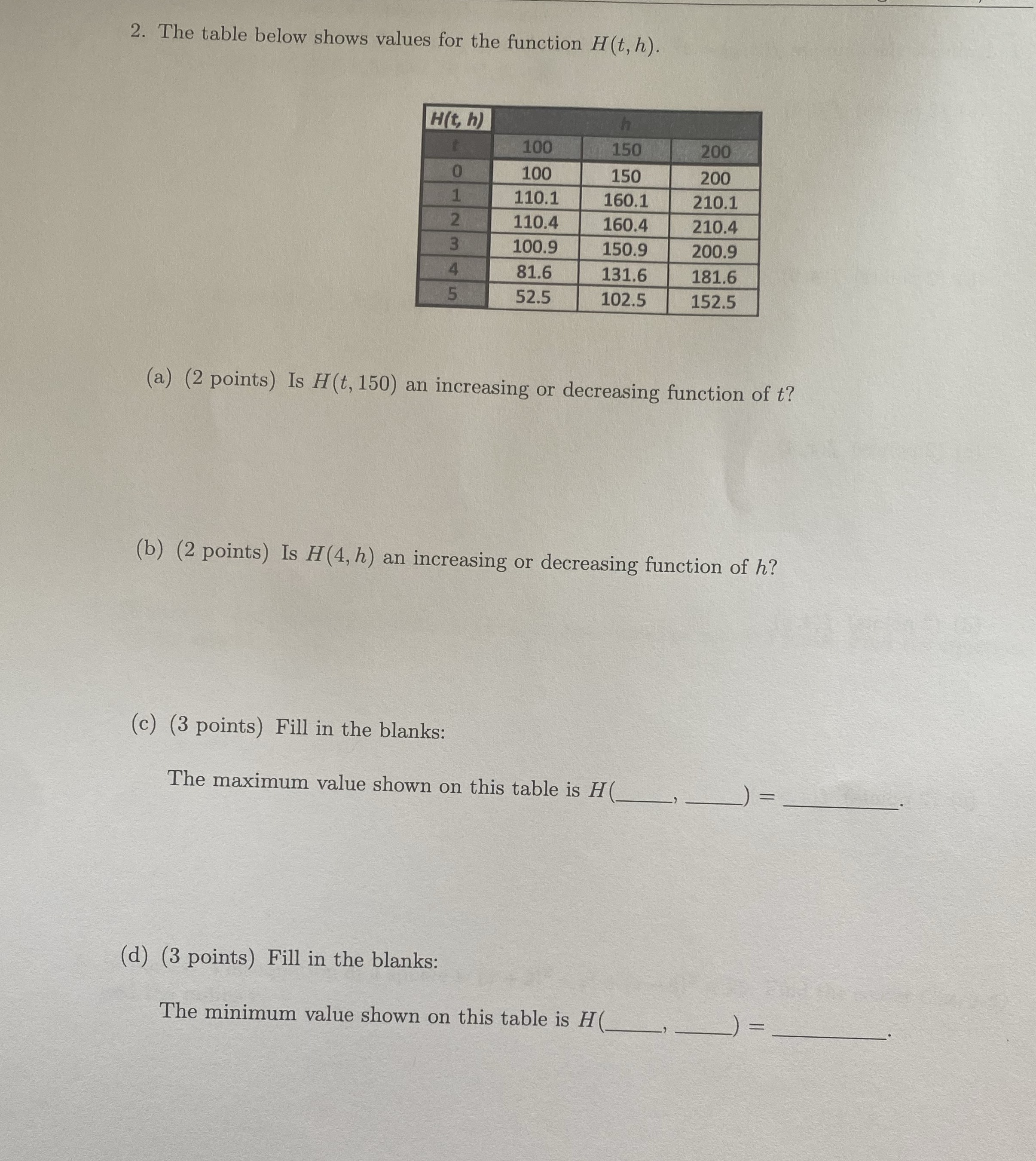### ¿Todavía tienes preguntas de matemáticas?

Pregunte a nuestros tutores expertos
Algebra
Pregunta2. The table below shows values for the function $$H ( t , h )$$ .

(a) (2 points) Is $$H ( t , 150 )$$ an increasing or decreasing function of $$t ?$$

(b) (2 points) Is $$H ( 4 , h )$$ an increasing or decreasing function of $$h ?$$

(c) (3 points) Fill in the blanks: The maximum value shown on this table is $$H ( \square, \square )$$$$\square$$  .

(d) (3 points) Fill in the blanks: The minimum value shown on this table is $$H ( \square, \square )$$$$\square$$ .